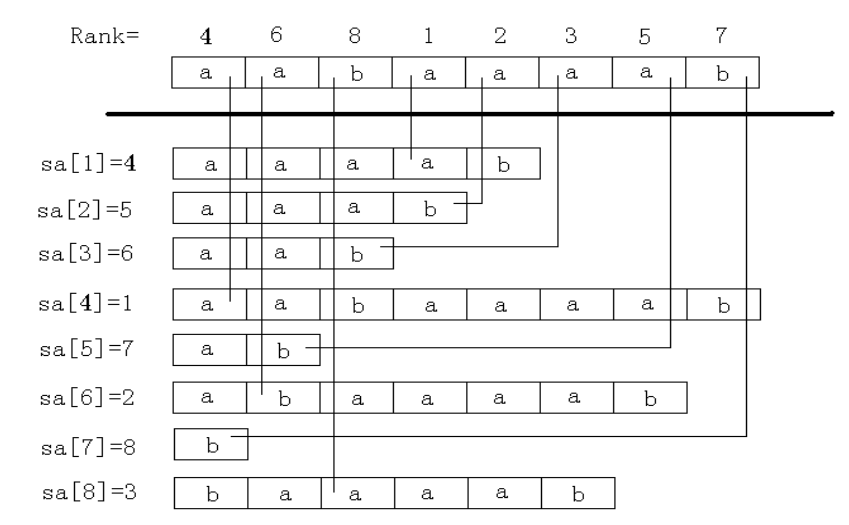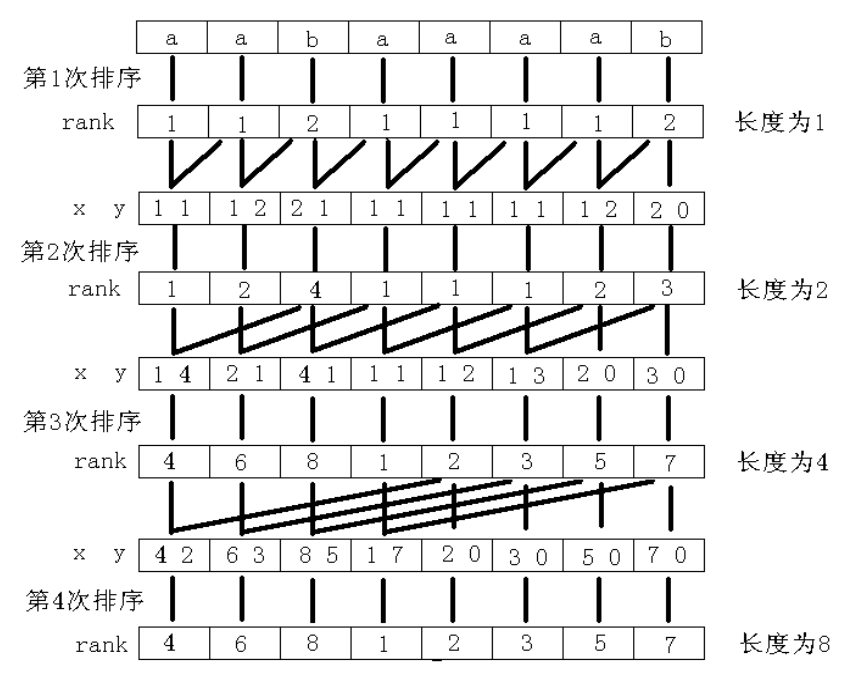## 简介

$rk[i]$ 代表后缀 $i$ 从小到大的排名。## 求法1 2 3 4 5 6 7 8 9 10 11 12 13 14 15 16 17 18 19 20 21 22 23 24 25 26 27 28 29 30  #include #include #include const int maxn = 1e6 + 100; char s[maxn]; int n, w, sa[maxn], rk[maxn << 1], oldrk[maxn << 1]; bool cmp(int a, int b) { if (rk[a] != rk[b]) return rk[a] < rk[b]; return rk[a + w] < rk[b + w]; } int main() { scanf("%s", s + 1); n = strlen(s + 1); for (int i = 1; i <= n; ++i) sa[i] = i, rk[i] = s[i]; for (w = 1; w < n; w <<= 1) { std::sort(sa + 1, sa + 1 + n, cmp); for (int i = 1; i <= n; ++i) oldrk[i] = rk[i]; //过程中需要用到原排名，因此拷贝一份 for (int i = 1, p = 0; i <= n; ++i) { if (oldrk[sa[i]] == oldrk[sa[i - 1]] && oldrk[sa[i] + w] == oldrk[sa[i - 1] + w]) rk[sa[i]] = p; //若两个子串相同，其排名需要相同 else rk[sa[i]] = ++p; } } for (int i = 1; i <= n; ++i) printf("%d ", sa[i]); return 0; } 

### 倍增基数排序

• 第二关键字无需计数排序。第一关键字相同时，先将 $i + w > n$ 的即第二关键字无穷小的元素放入队列中，再将普通元素放入。
• 优化计数排序的值域。每次对 $rk$ 数组进行了去重，因此可以不断缩小计数的值域。
• 用函数 $cmp$ 来计算是否重复，优化缓存。

• $sa[i]$ 表示排名第 $i$ 的后缀。
• $rk[i]$ 表示后缀 $i$ 的排名。
• $tp[i]$ 表示第二关键字排名为 $i$ 的后缀。
• $tax$ 为辅助基数排序所用的桶。
• $m$ 为当前计数排序的值域。

 1 2 3 4 5 6 7  void RadixSort() { for (int i = 1; i <= n; ++i) tax[i] = 0; for (int i = 1; i <= n; ++i) tax[rk[i]]++; //统计第一关键字排名为 i 的有多少个 for (int i = 1; i <= m; ++i) tax[i] += tax[i - 1]; //做前缀和方便得到全局排名 for (int i = n; i; --i) sa[tax[tp[i]]--] = tp[i]; //倒序遍历，保证在第一关键字相同的中，第二关键字靠后的排名靠后 } 

  1 2 3 4 5 6 7 8 9 10 11 12 13 14 15 16 17 18 19 20 21 22 23 24 25 26 27 28 29 30 31 32 33 34 35 36 37 38  #include #include #include const int maxn = 1e6 + 100; char s[maxn]; int n, M, sa[maxn], rk[maxn << 1], oldrk[maxn << 1], tax[maxn], tp[maxn]; void RadixSort() { for (int i = 1; i <= M; ++i) tax[i] = 0; for (int i = 1; i <= n; ++i) tax[rk[i]]++; //统计第一关键字排名为 i 的有多少个 for (int i = 1; i <= M; ++i) tax[i] += tax[i - 1]; //做前缀和方便得到全局排名 for (int i = n; i; --i) sa[tax[rk[tp[i]]]--] = tp[i]; //倒序遍历，保证在第一关键字相同的中，第二关键字靠后的排名靠后 } inline bool same(int a, int b, int w) { return oldrk[a] == oldrk[b] && oldrk[a + w] == oldrk[b + w]; } void SuffixArray() { M = 300; for (int i = 1; i <= n; ++i) rk[i] = s[i], tp[i] = i; RadixSort(); for (int w = 1, p; p < n; w <<= 1, M = p) //reset count range { p = 0; for (int i = n - w + 1; i <= n; ++i) tp[++p] = i; //第二关键字为无穷小的先放入 for (int i = 1; i <= n; ++i) if (sa[i] > w) tp[++p] = sa[i] - w; //初始化第二关键字 RadixSort(); for (int i = 1; i <= n; ++i) oldrk[i] = rk[i]; p = 0; for (int i = 1; i <= n; ++i) if (same(sa[i - 1], sa[i], w)) rk[sa[i]] = p; else rk[sa[i]] = ++p; } } int main() { scanf("%s", s + 1); n = strlen(s + 1); SuffixArray(); for (int i = 1; i <= n; ++i) printf("%d ", sa[i]); return 0; } 

• SA-IS
• DC3

{{{

}}}

{{{

}}}

“例题”

## height 数组

### height 数组定义

$height[i] = \operatorname{lcp}(sa[i-1],sa[i])$ .

$height$ 可以视为 $0$ .

### $O(n)$ 求 height 数组需要的一个引理

$$h[i] \ge h[i-1] - 1$$

\begin{aligned} & h[i] = \operatorname{lcp}(sa[rk[i] - 1],sa[rk[i]])\\ & h[i-1] = \operatorname{lcp}(sa[rk[i-1] - 1],sa[rk[i-1]) \\ \end{aligned}

$\operatorname{lcp}(sa[rk[i-1]-1]+1,sa[rk[i]]) = \min {height[k] \mid rk[sa[i-1]-1]+1 \le k \le rk[i]}$

$\operatorname{lcp}(sa[rk[i-1]-1]+1,sa[rk[i]]) \le h[i]$

$$h[i] \ge h[i-1] - 1$$

### $O(n)$ 求 height 数组的代码实现

 1 2 3 4 5 6 7 8 9  void initHeight() { for (int i = 1, k = 0; i <= n; ++i) { if (k) --k; while (s[i + k] == s[sa[rk[i] - 1] + k]) ++k; height[rk[i]] = h[i] = k; } } 

$k$ 最多减少 $n$ 次，最多加 $2n$ 次。

## height 数组的应用

• 子串是后缀的前缀

### 两子串最长公共前缀

$$\operatorname{lcp}(sa[i],sa[j]) = \min {height[i,\ldots,j]}$$

### 不同子串的数目

$$\dfrac{n(n+1)}{2} - \sum \limits _{i=2}^n height[i]$$